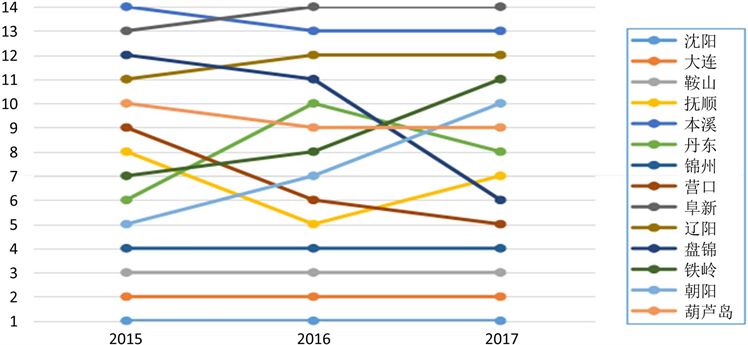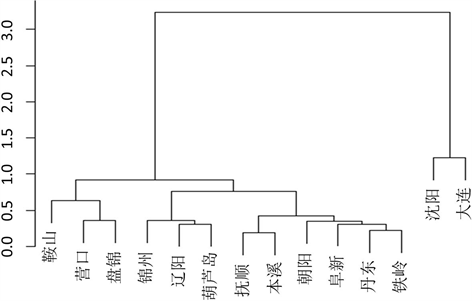# 基于因子分析的辽宁省城市综合竞争力评价分析Evaluation and Analysis of Comprehensive Competitiveness of Liaoning Province Based on Factor Analysis

DOI: 10.12677/AAM.2020.93051, PDF, HTML, XML, 下载: 170  浏览: 522  科研立项经费支持

Abstract: Liaoning has 14 prefecture-level cities, including Shenyang, Dalian and they play an important part in the whole country. Therefore, this thesis uses the factor analysis method, calculates the main factor score and the comprehensive score of 14 cities according to the relevant economic indicators, and gives the ranking. At the same time, 14 cities in Liaoning Province were classified and their pedigree maps were drawn by using the method of systematic clustering. It has an important reference value and a great guiding role for the local government in the economic regulation and control of the region.

1. 引言

2. 模型的介绍

2.1. 系统聚类

1) 首先，计算n个观测中所有 $\left(\begin{array}{l}n\\ 2\end{array}\right)=n\left(n-1\right)/2$ 对每两个数据之间的相异度(比如欧式距离)，将每个观测看作一类。

2) 令 $i=n,n-1,\cdots ,2$

i) 在i个类中，比较任意两类之间的相异度，找到相异度最小的(即最相似的)那一对，将他们结合起来。用两个类之间的相异度表示这两个类在谱系图中交汇的高度。

ii) 计算剩下的 $i-1$ 个新类中，每两个类之间的相异度。

2.2. 因子分析的理论介绍

$\left\{\begin{array}{l}{X}_{1}={a}_{11}{F}_{1}+{a}_{12}{F}_{2}+\cdots +{a}_{1m}{F}_{m}+{\epsilon }_{1}\\ {X}_{2}={a}_{21}{F}_{1}+{a}_{22}{F}_{2}+\cdots +{a}_{2m}{F}_{m}+{\epsilon }_{2}\\ \text{\hspace{0.17em}}\text{\hspace{0.17em}}\text{\hspace{0.17em}}\text{\hspace{0.17em}}\text{\hspace{0.17em}}\text{\hspace{0.17em}}\text{\hspace{0.17em}}\text{\hspace{0.17em}}\text{\hspace{0.17em}}\text{\hspace{0.17em}}\text{\hspace{0.17em}}\text{\hspace{0.17em}}\text{\hspace{0.17em}}\text{\hspace{0.17em}}\text{\hspace{0.17em}}\text{\hspace{0.17em}}\text{\hspace{0.17em}}\text{\hspace{0.17em}}\text{\hspace{0.17em}}\text{\hspace{0.17em}}\text{\hspace{0.17em}}\text{\hspace{0.17em}}\text{\hspace{0.17em}}\text{\hspace{0.17em}}\text{\hspace{0.17em}}\text{\hspace{0.17em}}\text{\hspace{0.17em}}\text{\hspace{0.17em}}\text{\hspace{0.17em}}\text{\hspace{0.17em}}⋮\\ {X}_{p}={a}_{p1}{F}_{1}+{a}_{p2}{F}_{2}+\cdots +{a}_{pm}{F}_{m}+{\epsilon }_{p}\end{array}$

$A=\left[\begin{array}{cccc}{a}_{11}& {a}_{12}& \cdots & {a}_{1m}\\ {a}_{21}& {a}_{22}& \cdots & {a}_{2m}\\ ⋮& ⋮& \ddots & ⋮\\ {a}_{p1}& {a}_{p2}& \cdots & {a}_{pm}\end{array}\right]$

1) $X={\left({X}_{1},{X}_{2},\cdots ,{X}_{p}\right)}^{\prime }$ 是可观测随机向量，且 $E\left(X\right)=0$$\mathrm{cov}\left(X\right)=\Sigma$，且 $\Sigma$ 等于相关矩阵R。

2) $F={\left({F}_{1},{F}_{2},\cdots ,{F}_{m}\right)}^{\prime }\left(m 是不可观测的变量， $E\left(F\right)=0$$\mathrm{cov}\left(F\right)=I$，即向量F的各个分量是互相独立的。

3) $\epsilon ={\left({\epsilon }_{1},{\epsilon }_{2},\cdots ,{\epsilon }_{p}\right)}^{\prime }$ 与F相互独立，且 $E\left(\epsilon \right)=0$$\epsilon$ 的协方差矩阵 ${\Sigma }_{\epsilon }$ 是对角阵：

$\mathrm{cov}\left(\epsilon \right)={\Sigma }_{\epsilon }=\left[\begin{array}{cccc}{\sigma }_{11}^{2}& & & 0\\ & {\sigma }_{22}^{2}& & \\ & & \ddots & \\ 0& & & {\sigma }_{pp}^{2}\end{array}\right]$

$\epsilon$ 的各分量之间也是互相独立的。

$A=\left(\sqrt{{\lambda }_{1}}{\gamma }_{1},\sqrt{{\lambda }_{2}}{\gamma }_{2},\cdots ,\sqrt{{\lambda }_{m}}{\gamma }_{m}\right)$

3. 建立模型与分析结果

3.1. 数据的预处理

3.2. 因子分析法分析结果

Table 2. Load matrix after factor rotationTable 3. Contribution rate of main factors and cumulative contribution rateTable 4. City comprehensive competitiveness scores and rankingsTable 5. Comprehensive scores and rankings of cities in Liaoning in 2015 and 2016Figure 1. Line chart of competitiveness ranking of cities in Liaoning from 2015 to 2017

3.3. 系统聚类分析结果Figure 2. System clustering pedigree

4. 结论

  张晨瑶. 东北三省城市竞争力评价研究[J]. 中国国情力, 2019(5): 47-51  熊妮君, 黄慧妍, 肖雄方, 陈虹桔, 戴洁琳. 比较视角下的广西城市竞争力研究[J]. 现代经济信息, 2019(2): 489-491.  黄国妍, 刘江会, 姜鑫涛, 贾高清. 基于复杂网络分析方法的国内外主要城市竞争力比较研究[J]. 城市发展研究, 2019, 26(1): 38-46.  刘林军, 吴黎军. 基于因子分析的我国西部12城市经济发展状况实证分析[J]. 重庆理工大学学报(自然科学版)， 2010, 24(11): 118-122.  马丽娜. 基于因子分析的陕西各城市综合经济实力分析[J]. 电脑知识与技术:学术交流, 2018(12Z): 256-258.  郑阳. 基于主成分分析的辽宁省城市竞争力评价[D]: [硕士学位论文]. 长春: 吉林大学, 2011.  李娟文, 王启仿. 中国副省级城市经济可持续发展能力差异综合评价[J]. 经济地理, 2001, 21(6): 665-668.  李超楠. 几种山东省GDP的预测方法及其比较[D]: [硕士学位论文]. 济南: 山东大学, 2018.